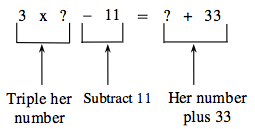### Home > MC2 > Chapter 1 > Lesson 1.2.2 > Problem1-66

1-66.

Alice said, " I am thinking of a number. If you triple my number and subtract $11$, you get my number plus $33$. What's my number?" Find Alice's number. Show how you know your answer is correct.

Use the Guess and Check method.Use different values where the question marks are until you get the right answer.

Alice's number is between $20$ and $30$.